# MLP-Mixer An all-MLP Architecture for Vision

Google Brain proposed MLP-Mixer (code is available in google-research/vision_transformer official) which solely used multi-perceptron network (MLP) for computer vision tasks. This is different most commonly used convolution neural network (CNN) or more recently transformer based approaches. The experiment on image classification indicates that, given sufficient amount of data (e.g., 100M images) for pre-training then fine-tuned for target task (ImageNet 2012), MLP-Mixer is able to achieve competitive result as CNN and transformer. However, the performance drops far belower than CNN when insufficient amount of data are available for pre-training, especially for its larger variation. It is also found at similar accuracy, MLP-Mixer and transformer are faster than CNN (ResNet) for inference and training by 2~3 times.

Image below shows the an overview of the proposed model.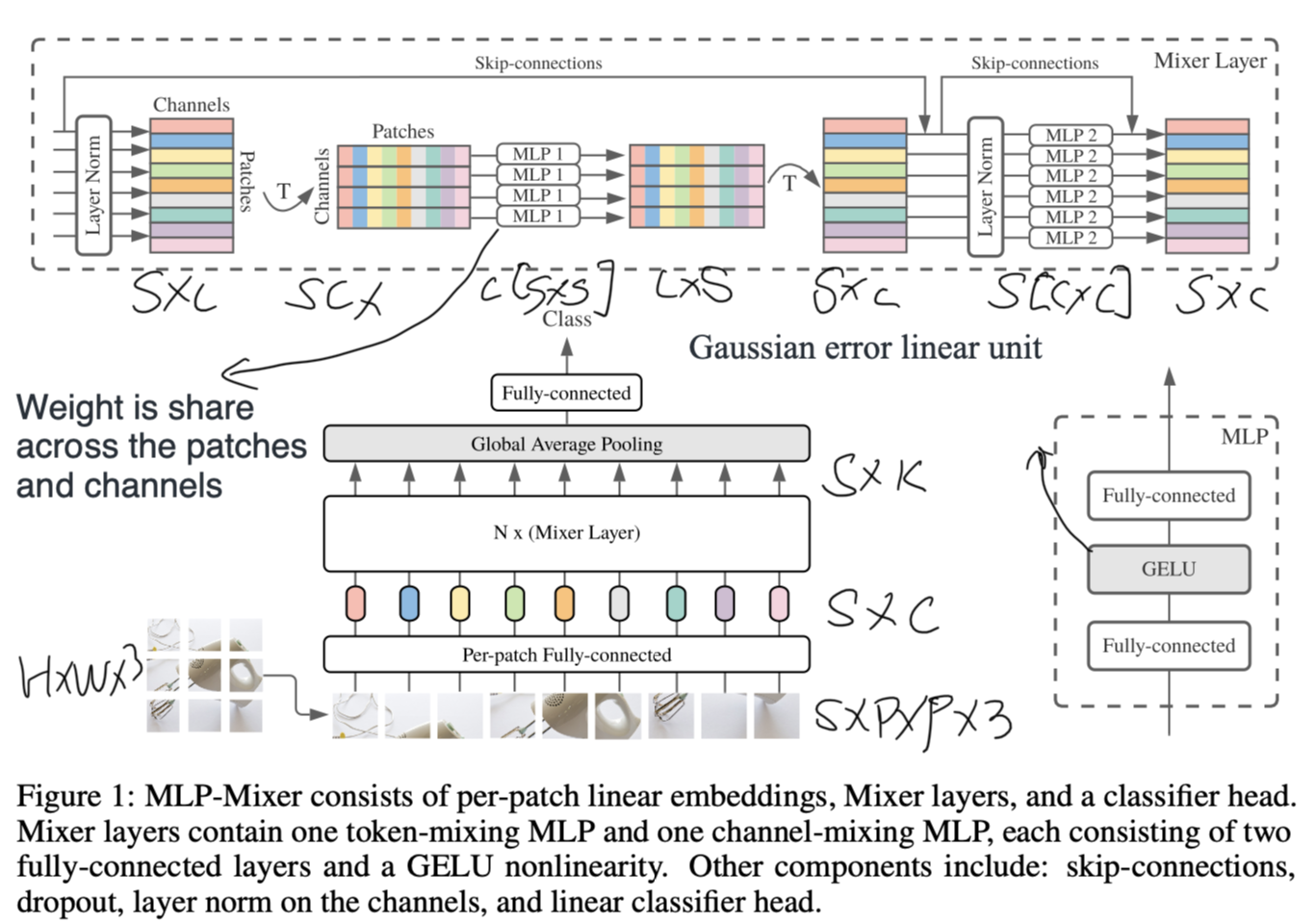# Proposed Method

It accepts a sequence of linearly non-overlaping projected image patches (also referred to as tokens) shaped as a “patches × channels” table as an input, and maintains this dimensionality. Here assume the input image is $$H\times W \times 3$$ and divided into patches $$S\times P\times P \times 3$$, where $$S=\frac{H}{P}\frac{W}{P}$$ is the number of patches. Each patch is projected to a feature vector of $$\mathbb{R}^{C\times 1}$$ via a fully connected layer shared for all patches.

Mixer makes use of two types of MLP layers: channel-mixing MLPs and token-mixing MLPs:

• The channel-mixing MLPs allow communication between different channels; they operate on each token independently and take individual rows of the table as inputs.
• The token-mixing MLPs allow communication between different spatial locations (tokens); they operate on each channel independently and take individual columns of the table as inputs.

These two types of layers are interleaved to enable interaction of both input dimensions. The MLP layers could be written as:

$U_{,i}=X_{,i}+W_2\sigma(W_1\mbox{LayerNorm}(X)_{,i})\mbox{ for }i=1,...,C\\ Y_{j,}=U_{j,}+W_4\sigma(W_3\mbox{LayerNorm}(U)_{j,})\mbox{ for }j=1,...,S$

Especially:

• the channel-mixing MLPs share the same weight across patches and the token-mixing MLPs share the weight across channels. The ablation study indicates that unsharing the weights cross channels or only sharing weight in group of channels does’t improve the accuracy.
• the size of data (number of patches and channels) doesn’t change in the network. While first experiments showed that on JFT-300M such models significantly reduced training time without losing much performance, we were unable to transfer these findings to ImageNet or ImageNet-21k

# Experiment Result

## Implementations

We pre-train all models using Adam with $$\beta_1= 0.9$$, $$\beta_2= 0.999$$, and batch size 4096, using weight decay, and gradient clipping at global norm 1. We use a linear learning rate warmup of 10k steps and linear decay. We pre-train all models at resolution 224. The following data augmentation technique is applied: cropping, random horizontal flip, RandAugment, mixup, dropout and stochastic depth.

We fine-tune using SGD with momentum, batch size 512, gradient clipping at global norm 1, and a cosine learning rate schedule with a linear warmup. We do not use weight decay when fine-tuning.

## Experiment Result

### Accuracy over Pre-Training Size

The table above compares the performance of MLP-Mixer method (pink) to transformer (blue) and CNN. The result are very close which is within 1% for top-1 accuracy, when sufficient amount of data (e.g., 10s of million) is available for pre-training. Note 1.2M images from ImageNet 2012 alone is insufficient.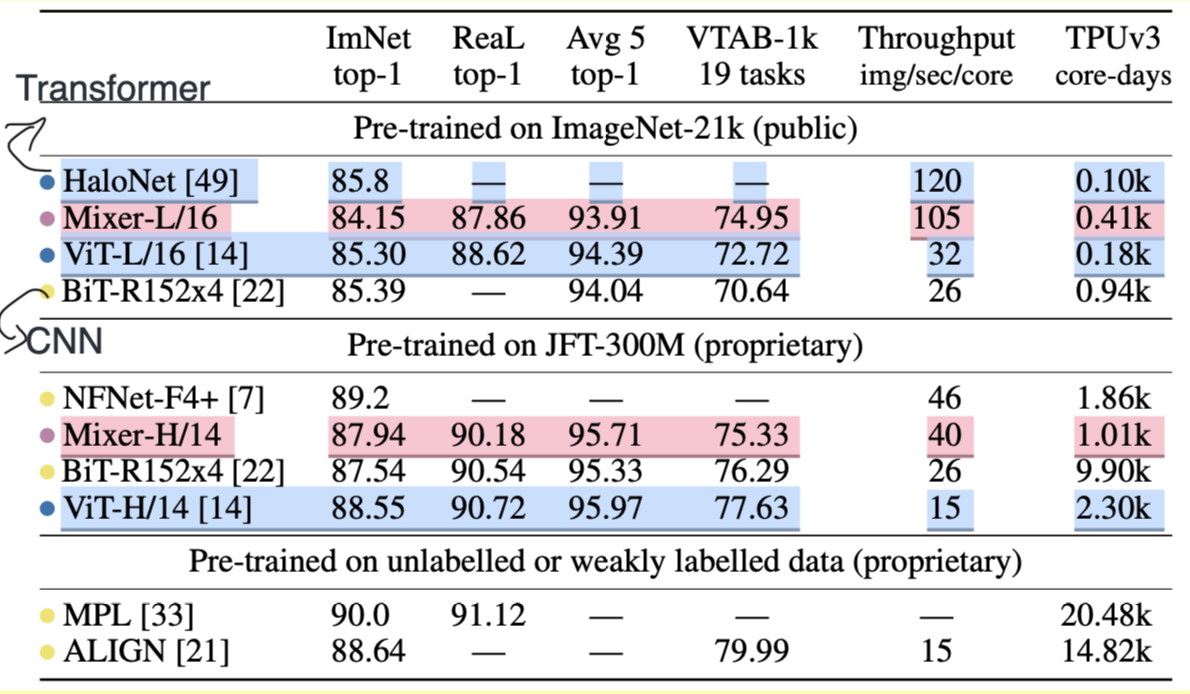This figure illustrates the accuracy in terms of pre-training size. Obviously CNN (black dot curve) is outperforms the transformer and MLP-mixer when <100M images are available for pre-training; as more than 100M images are available for pre-training, the bigger variants of MLP-mixer and transform begin to outperform CNN.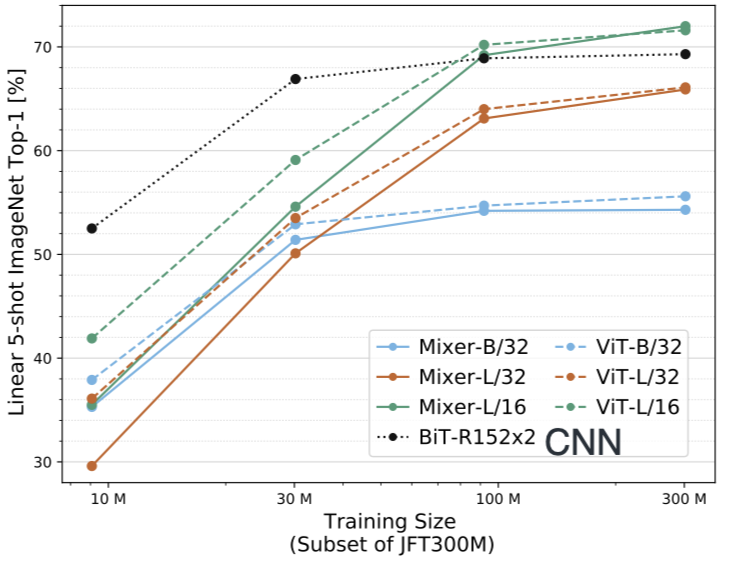### Training and Inference Time

Those figures the training time and inference time. It indicates that, at similar accuracy, MLP-Mixer and transformer are faster than CNN (ResNet) for inference and training by 2~3 times.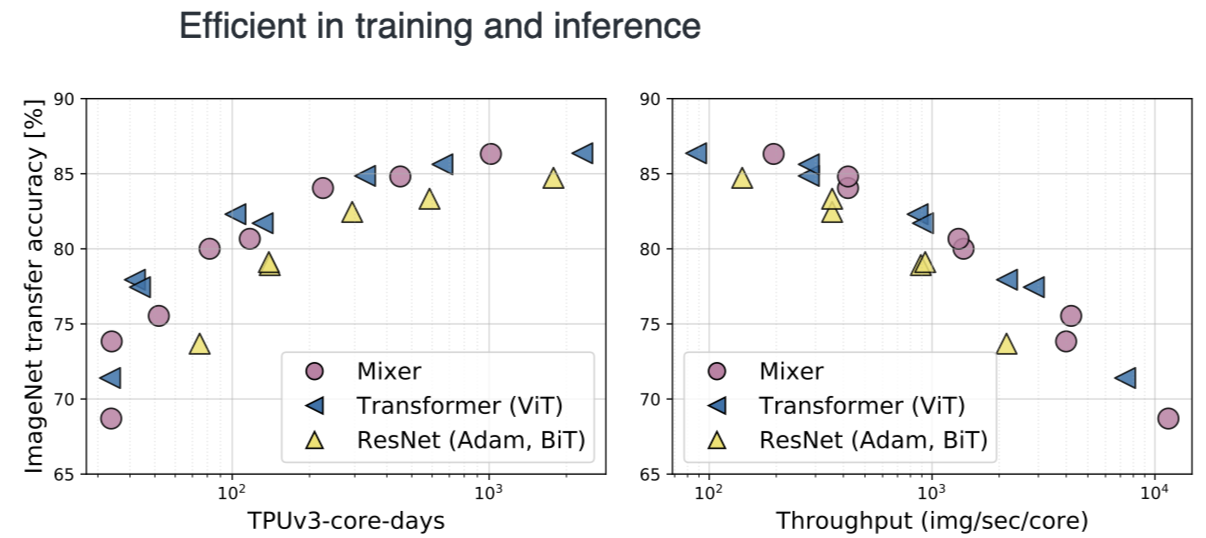### Accuracy vs Model Size

This table compares the performance of model vs mode size. Obviously, when only ImageNet 2012 (1.2M images) is avaiable for training and pre-training, the accuracy of MLP-Mixer is low. The larger variant (L/16) suffers more than the smaller variant (B/16)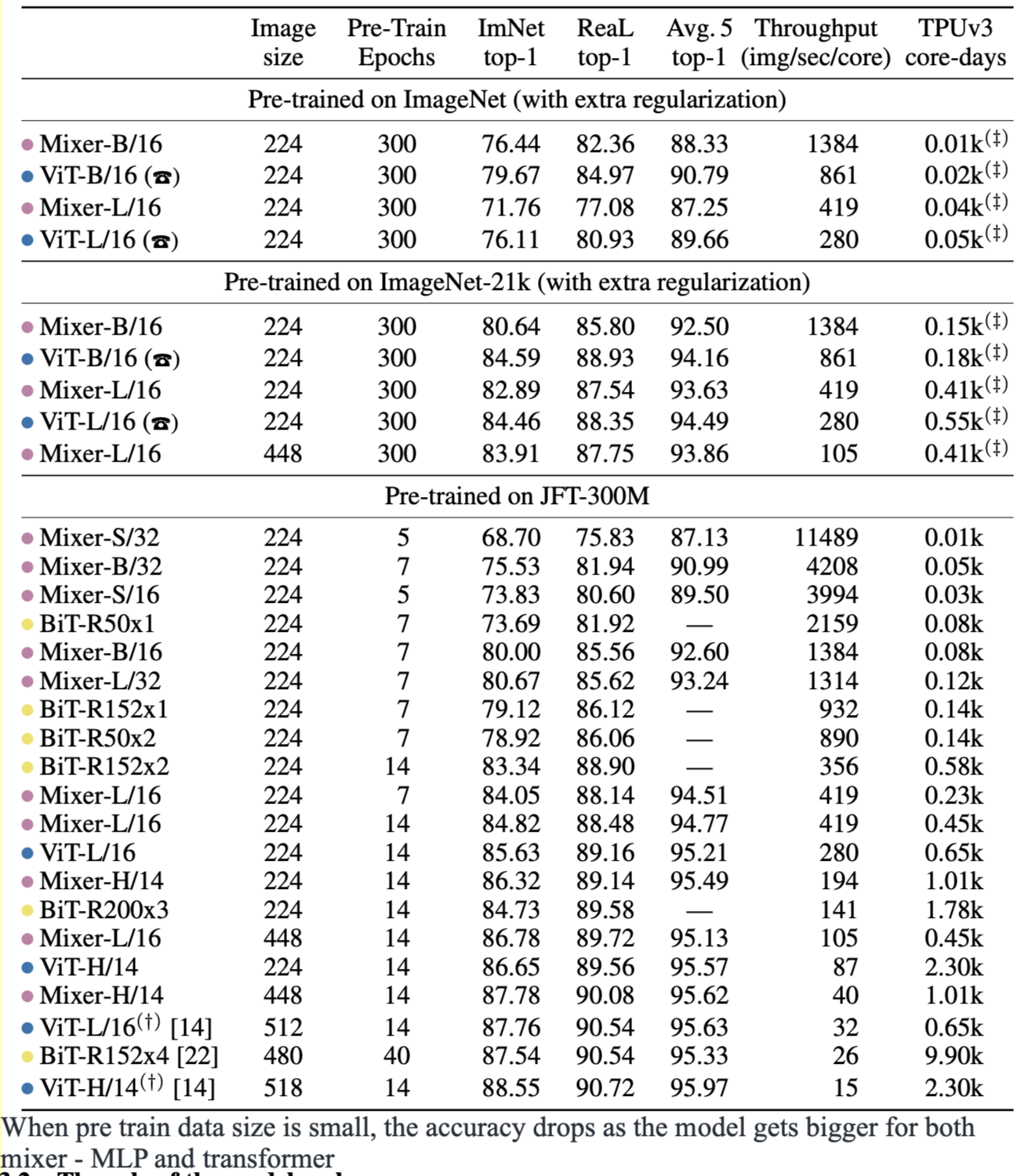# Relationship to CNN and Transformer

Modern deep vision architectures consist of layers that mix features (i) at a given spatial location, (ii) between different spatial locations, or both at once. In CNNs, (ii) is implemented with N × N convolutions (for N > 1) and pooling. Neurons in deeper layers have a larger receptive field [1, 27]. At the same time, 1×1 convolutions also perform (i), and larger kernels perform both (i) and (ii). In Vision Transformers and other attention-based architectures, self-attention layers allow both (i) and (ii) and the MLP-blocks perform (i). The idea behind the Mixer architecture is to clearly separate the per-location (channel-mixing) operations (i) and cross-location (token-mixing) operations (ii). Both operations are implemented with MLPs.

When we compare the MLP-Mixer shares to CNN, we could find some interesting points:

• channel-mixing MLP is very similar to convolution layer with $$1\times1$$ kernel size. It is not uncommon operator;
• token-mixing MLP could be viewed as channel separable convolution with whole patch as kernel size. Though typically CNN now uses 3x3 as kernel size, but early CNN did use 7x7 or even 11x11 kernel size, e.g., AlexNet
• in CNN, the patch, though not explicitly defined, is used and extracted from images with overlap, which is controlled by stride parameter. Kernel size is equal to patch size. Instead MLP-mixer explictly extract patches with non-overlap or stride = kernel size;
• in both MLP-Mixer and CNN, the pixels within the same pixels are interacted to each other. But in MLP-Mixer this is explicitly performed in first fully connected layer; CNN does this at every convolution layer.
• CNN relies on pooling layer to gradually interact pixels cross patches or at longer distance. Thus CNN’s output feature map’s resolution is usually much lower than input resolution (e.g., $$\frac{1}{32}\times\frac{1}{32}$$). In MLP-Mixer, all patches interact to all others in each token-mixing MLP of each MLP layer. MLP-Mixer tends to keep the resolution through the network.
Written on May 8, 2021